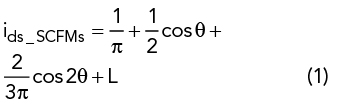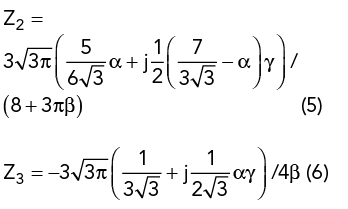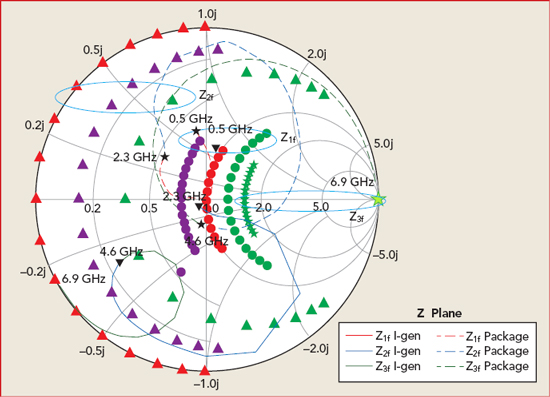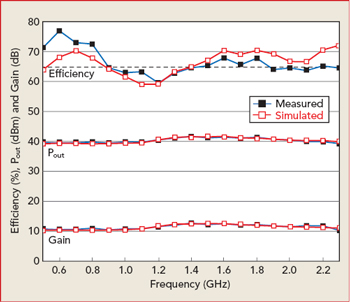# Broadband Power Amplifier Design Using Extended Resistive-Reactive Continuous Class F Modes

June 13, 2019

This article describes an approach to extend the bandwidth of power amplifiers (PA) designed using resistive-reactive series of continuous class F modes (SCFM). By introducing third harmonic loads to the resistive-reactive SCFM PA, the overlap between the fundamental and harmonic impedances is solved and the operating bandwidth extended. Using this approach, a 0.5 to 2.3 GHz high efficiency PA was designed, with experimental results achieving 10 W output power and 59 to 79 percent drain efficiency from 0.5 to 2.3 GHz.

With the rapid development of wireless communications technology, next-generation wireless systems need wider bandwidth to achieve higher data rate transmission. As a key transmitter element, PAs are required to work more efficiently, over broader bandwidths and handle multiple standards.

In recent years, much research has explored improving the bandwidth and efficiency of PAs. In 2009, S. C. Cripps proposed the continuous mode PA to solve the bandwidth limitation of traditional switch-mode PAs, by properly introducing reactive second and third harmonic impedances.1 Continuous class B/J, continuous class F and class F1 modes were subsequently proposed.2-6 In theory, the maximum bandwidth of continuous class B/J, continuous class F and class F1 PA modes is restricted to an octave due to harmonic impedances at the edge of the Smith chart. Thus, the rigorous requirements of harmonic loads created difficulty realizing multi-octave performance. The resistive-reactive series of continuous modes was proposed by Lu and Chen in 20137 to relax the strict demands of harmonic loads, introducing resistive harmonic impedances to the continuous modes.8-9 With this approach, the bandwidth can exceed an octave by introducing resistive second harmonic loads and a more extensive fundamental impedance space, extending the bandwidth of broadband PAs. The resistive-reactive series of inverse continuous modes PA proposed by Li et al.9 illustrates similar properties for the design of broadband PAs.

In this article, an extended mathematical formula is applied to the resistive-reactive SCFM. The design space is further extended by introducing third harmonic impedances, providing more freedom when designing high efficiency multi-octave PAs.

Extended Resistive-Reactive SCFM

The traditional resistive-reactive SCFM has a half-wave rectified sinusoidal current waveform, ids(θ), at the intrinsic current generator plane of the device:The voltage waveform, vds (θ), is no longer rigorously restricted to a square waveform and includes a set of variations dependent on the parameters α and γ:Resistive third harmonic impedances are introduced by multiplying the current waveform of the resistive-reactive SCFM by the factor (1+β cos θ), while the voltage waveform remains unchanged. The new current waveform is represented by:Alternative impedance solutions with resistive second and third harmonic impedances can be obtained. The load impedance to be presented at each harmonic is calculated by dividing the voltage by the current. Here, Zn is designated as the nth-order harmonic impedance. Thus, the normalized harmonic impedances can be calculated from:The feasibility of Z1, Z2 and Z3 depends on the conditions

0 ≤ α ≤ 1 and −8/3π ≤ β ≤ 0

being fulfilled. Figure 1 illustrates the variation of the fundamental and harmonic impedances vs. α and β. The second harmonic region moves toward the fundamental region with variation of α and β, and the third harmonic region approaches the fundamental region with decreasing β. This characteristic makes solving the overlap between the fundamental and harmonic impedances in a multi-octave design possible.Figure 1 Fundamental and harmonic impedances vs. α and β.

Drain efficiency can be calculated from Equations 2 and 3 as:Drain efficiency is a function of α and β. The variation of drain efficiency and output power with respect to α and β are shown in Figure 2. The variation of α and β should be restricted within a limited region to achieve acceptable drain efficiency with a slight degradation of output power. In this work, the conditions of 0 ≤ α ≤ 0.4 and −0.4 ≤ β ≤ 0 are selected to achieve drain efficiency greater than 65 percent.Figure 2 Drain efficiency (a) and normalized output power (b) vs. α and β.

Simulation and Measurement

To verify the effectiveness of this approach, a 0.5 to 2.3 GHz resistive-reactive SCFM PA was designed using a Wolfspeed CGH40010F GaN transistor, operating at a quiescent drain bias of 28 V and of 68 mA, and a 30 mil thick Rogers 4350B substrate (εr = 3.66) with a conductor thickness of 35 μm.

The optimum load impedances were obtained by harmonic load-pull simulation using an iterative process from high to low frequency, where the impedance obtained at the high frequency was used to terminate the low frequency harmonic. This was repeated until the optimum load impedances were obtained. The output matching network was designed using the real frequency direct computational technique.10 Figure 3 shows the broadband output matching network for this design. Due to the small effect of input harmonic impedance on performance,11 when designing the input matching network, more attention was paid to fundamental matching.Figure 3 Output matching network.

A precise model of the parasitic network for the widely used CGH40010F transistor has been derived and reported by Tasker and Benedikt.12 Based on this parasitic network model, the impedance trajectories in the Smith chart at I-gen and at the package plane of the output matching network are shown in Figure 4. The derived fundamental impedances at the current plane across the operating band from 0.5 to 2.3 GHz are in or near the theoretic region.Figure 4 Fundamental and harmonic impedances at the I-gen and package planes.

The final design of the resistive-reactive SCFM PA is shown in Figure 5. Simulated and measured results with a CW input power of 29 dBm are shown in Figure 6. A drain efficiency of 59 to 79 percent and saturated output power of 39.4 to 41.6 dBm are achieved across the band from 0.5 to 2.3 GHz. The measured results are consistent with the simulations.Figure 5 PA schematic (a) and prototype (b).Figure 6 Simulated vs. measured output power, efficiency and gain.Figure 7 Measured average drain efficiency and ACLR with 20 MHz LTE signal.

To characterize linearity, a 20 MHz LTE signal with a peak-to-average power ratio of about 7.5 dB was used to drive the PA at 0.8, 1, 1.6 and 2 GHz. As shown in Figure 7, the broadband PA exhibits good linearity at about 5 dB back-off power, where the adjacent channel leakage power ratio (ACLR) is lower than −30 dBc, with 34.1 to 49.1 percent average efficiency. Table 1 compares the performance of this PA with other similar state-of-the-art broadband PAs.

CONCLUSION

The resistive-reactive SCFM design space was extended by introducing the third harmonic impedance. Using this methodology, the overlap between the fundamental and harmonic impedances was effectively solved, and a broadband high efficiency PA was designed, built and tested. The agreement between simulation and measurement demonstrates the validity of this approach for a multi-octave, high efficiency PA. Driven by 20 MHz LTE signals, the ACLR of the proposed PA is lower than −30 dBc at approximately 35 dBm output power, with average drain efficiency higher than 34 percent.

Acknowledgments

This research was supported by the Natural Science Foundation of China under grant 61501455, Natural Science Foundation of Beijing Municipality under grant 4162068 and National Defense and Technology Innovation Foundation of Chinese Academy of Sciences under grant CXJJ-16M124.References

1. S. C. Cripps, P. J. Tasker, A. L. Clarke, J. Lees and J. Benedikt, “On the Continuity of High Efficiency Modes in Linear RF Power Amplifiers,” IEEE Microwave & Wireless Components Letters, Vol. 19, No. 10, October 2009, pp. 665–667.
2. P. Wright, J. Lees, J. Benedikt, P. J. Tasker and S. C. Cripps, “A Methodology for Realizing High Efficiency Class-J in a Linear and Broadband PA,” IEEE Transactions on Microwave Theory & Techniques, Vol. 57, No. 12, December 2009, pp. 3196–3204.
3. J. Chen, S. He, F. You, R. Tong and R. Peng, “Design of Broadband High-Efficiency Power Amplifiers Based on a Series of Continuous Modes,” IEEE Microwave & Wireless Components Letters, Vol. 24, No. 9, September 2014, pp. 631–633.
4. V. Carrubba, J. Lees, J. Benedikt, P. J. Tasker and S. C. Cripps, “A Novel Highly Efficient Broadband Continuous Class-F RFPA Delivering 74% Average Efficiency for an Octave Bandwidth,” IEEE MTT-S IMS Digest, June 2011.
5. V. Carrubba, A. L. Clarke, M. Akmal, Z. Yusoff, J Lees, J. Benedikt, S. C. Cripps and P. J. Tasker, “Exploring the Design Space for Broadband PAs Using the Novel ‘Continuous Inverse Class-F Mode’,” 41st European Microwave Conference, October 2011.
6. W. Shi, S. He and Q. Li, “A Series of Inverse Continuous Modes for Designing Broadband Power Amplifiers,” IEEE Microwave & Wireless Components Letters, Vol. 26, No. 7, July 2016, pp. 525–527.
7. Z. Lu and W. Chen, “Resistive Second-Harmonic Impedance Continuous Class-F Power Amplifier with Over One Octave Bandwidth for Cognitive Radios,” IEEE Journal on Emerging & Selected Topics in Circuits & Systems, Vol. 3, No. 4, December 2013, pp. 489–497.
8. C. Friesicke, R. Quay and A. F. Jacob, “The Resistive-Reactive Class-J Power Amplifier Mode,” IEEE Microwave & Wireless Components Letters, Vol. 25, No. 10, October 2015, pp. 666–668.
9. Q. Li, S. He, Z. Dai and W. Shi, “A Method for Designing Generalized Continuous Power Amplifier,” IEEE MTT-S International Microwave Workshop Series on Advanced Materials and Processes for RF and THz Applications, July 2016.
10. N. Tuffy, L. Guan, A. Zhu and T. J. Brazil, “A Simplified Broadband Design Methodology for Linearized High Efficiency Continuous Class-F Power Amplifiers,” IEEE Transactions on Microwave Theory & Techniques, Vol. 60, No. 6, March 2012, pp. 1952–1963.
11. J. Moon, J. Kim and B. Kim, “Investigation of a Class-J Power Amplifier With a Nonlinear Cout for Optimized Operation,” IEEE Transactions on Microwave Theory & Techniques, Vol. 58, No. 11, November 2010, pp. 2800–2811.
12. P. J. Tasker and J. Benedikt, “Waveform Inspired Models and the Harmonic Balance Emulator,” IEEE Microwave Magazine, Vol. 12, No. 2, April 2011, pp. 38–54.